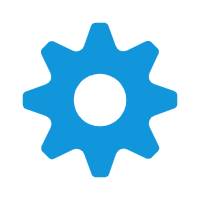Title# C++初学之LeetCode刷题基础

2019/5/13

个人说明：这是我第一次写博客文章，主要是试写，写的不好请谅解。

### 初学C++

##### 下面是关于函数部分的学习
1. 内联函数
2. 默认参数列表
3. 缺省参数列表
4. 哑元函数

1. 内联函数

函数前面加上inline 关键字

###### 1.内联函数调用原理

1.内联函数与宏定义的区别
C语言使用预处理器语句#define来提供宏。如下例所示：
#define SQUARE(X) X*X

a = square(5.0);->a=5.0*5.0;
b = square(4.5+7.5);->b=4.5+7.5*4.5+7.5
d = square(c++);->d=c++*c++

#define SQUARE(X) ((X)*(X))

2.默认参数列表：

1 int info(int a=10,int b=20,int c=30)
2 {
3     return a+b+c;
4 }

 1 void foo(char a='b',int b=2,const char* c="xY")
2 {
3      std::cout<<a<<","<<b<<","<< c<<endl;
4 }
5
6 /*
7
8   注意这里的参数必须写满
9
10 */

### 3.缺省参数列表

 1 void bar(char a,int b,const char*c="XY")
2 {
3     cout<<a<<","<<b<<","<<c<<endl;
4 }
5
6 /*
7
8    你的参数如果缺省，从前面开始缺，后面的默认参数要连在一起
9
10
11 */

void bar(char a='b',int b,const char*c)
{
cout<<a<<","<<b<<","<<c<<endl;
}

/*
记忆与理解：
由于参数是从左边到右边进行匹配的。看下面，调用bar(3,"4"),这样 3就赋值给 字符a,

中间的b,和 c怎么分配？
因此，规定，默认参数从右边往左边写

*/

int main(void)
{

bar(3,"4");

}

### 4.哑元函数

void func(int,int b,int)
{
cout<<b<<endl;

}

int main(void)
{
func(1,2,3);

//传参一个都不能少，必须和参数申明的个数一样

}

posted @ 2019-05-13 22:44  .geek  阅读(2191)  评论(0编辑  收藏  举报